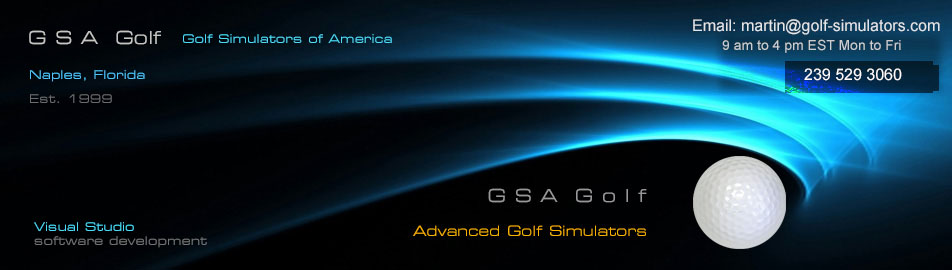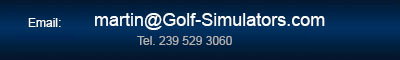Club Tracking Camera Mono Club Tracking Camera Color Ball spin camera Line Scan camera Stereo Cameras Camera Wiring Camera Installation Installation and setup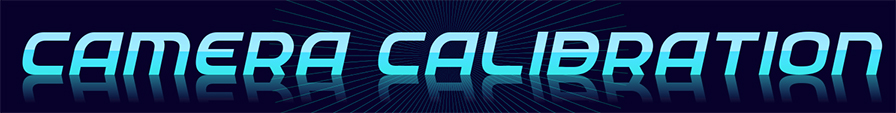V and H cam calibration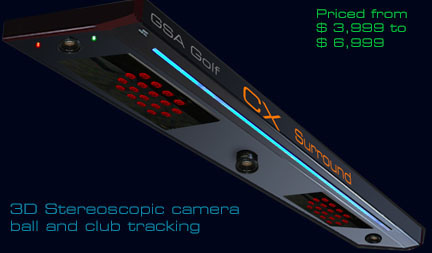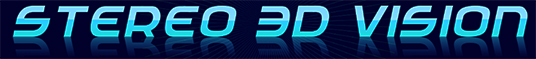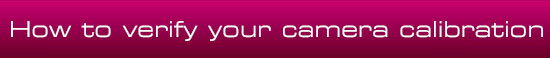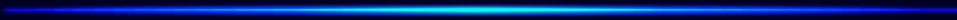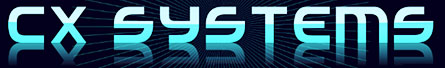-• The primary purpose of calibration is to ensure that ball speeds and launch angles are measured accurately.
• The calibration method here is to use a white tube of a known length on a pole that represents a ball trace at a constant speed which
• is then placed at various vertical and horizontal angles.
• The object of the calibration is to ensure that the length of the trace (i.e. white tube) remains constant at all angles and the launch angle remains
• constant for the particular tilt of the pole as measured by an inclination meter
• Note: Please ensure you're using CP version 8.6.3.5 or higher for these instructions
• Also note that these calibration and adjustment methods are intended for advanced users only
• as most are ok with the basic default settings and calibration as supplied with the systems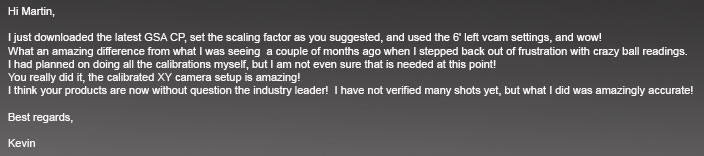Above, a customer e-mail from 04/21/2017 regarding the new extended calibrationFactory calibration table set

All current GSA Golf Control Panel versions and updates now include a factory set camera extended calibration table

that will be used if users choose not to calibrate the cameras themselves.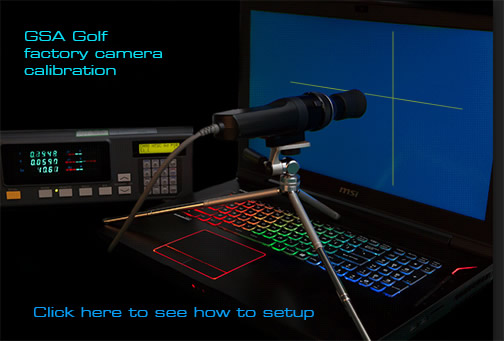see the end of this page for factory calibration details or click the above image

XY camera setup calibration

Why is calibration or correction required?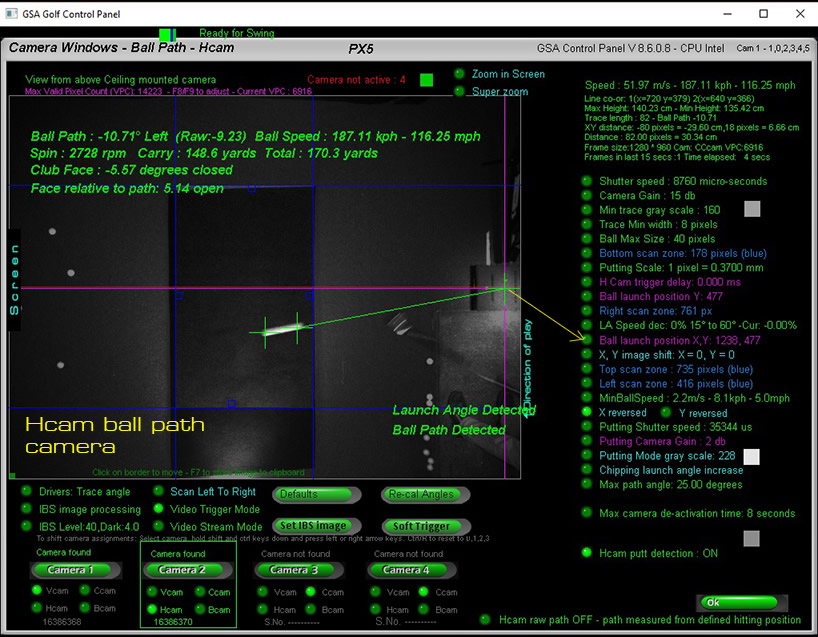With the classic XY camera setup, the Hcam is mounted on the ceiling looking straight down to see the direction of the ball left and right (as shown in the above image)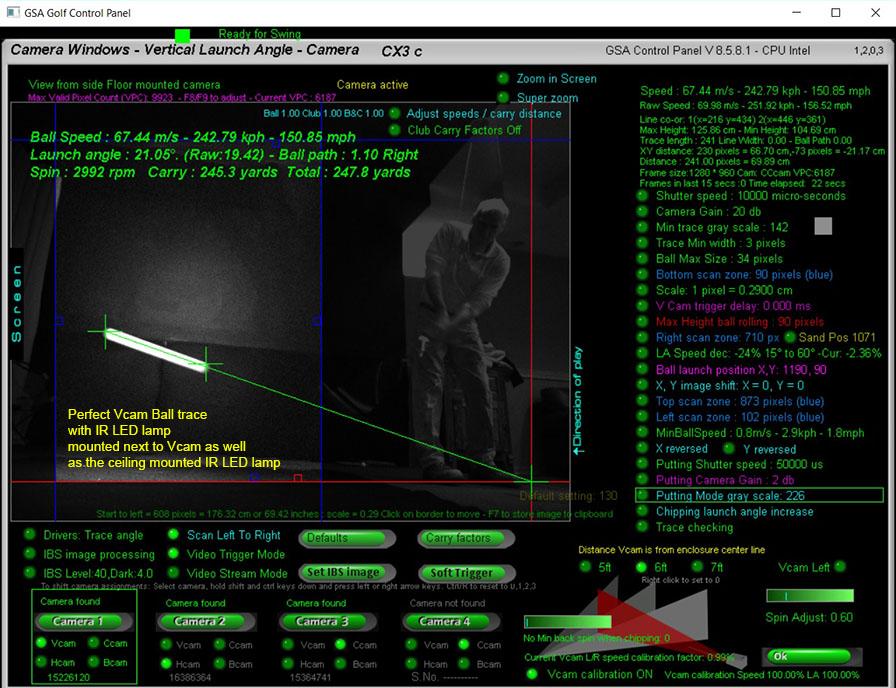• and the Vcam is mounted at floor level either on the left or right hand side of the enclosure and views the ball in flight from the side.
• (as shown in the above image)
• Note that the Vcam - in addition to measuring the vertical launch angle of the ball - also measures the speed of the ball based on the length of the ball trace.
• i.e. The length of the trace is directly proportional to the speed of the ball.
• As long as the ball is hit perfectly straight and low, this setup wouldn't require any calibration.
• Ball speed and launch angle (LA) accuracy problems occur when the ball is hit either left or right or higher.
• This is due to the ball being hit either further away or nearer to the cameras which causes the ball trace images to appear either larger or smaller.
• If not adjusted this ball trace size difference will distort the speed measurements.
• eg if the Vcam is mounted on the right side and the ball is hit to the right then the ball trace image will appear larger in the camera and thus the ball speed will
• be measured higher than it actually is.
• Likewise, if the ball is hit to the left away from the Vcam camera then the ball trace image will appear smaller and thus the ball speed will be measured lower
• than it actually is.

Two methods of correction

The two methods of correction are:

1. Full calibration from a pre-defined or user defined calibration table

2. A user defined percentage correction

Full calibration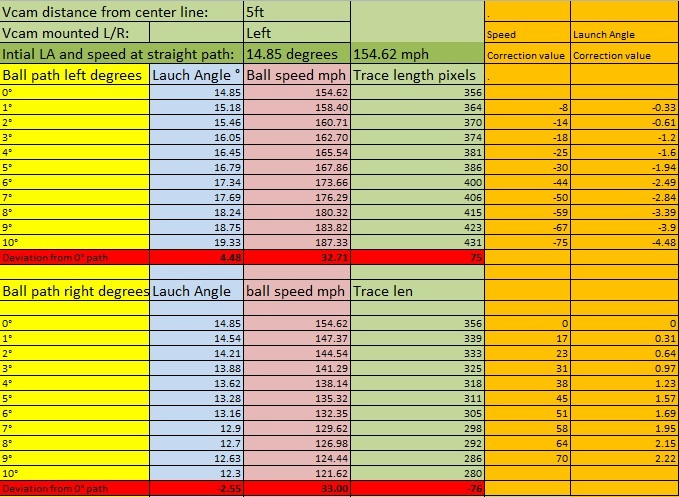• The full calibration method uses pre-defined calibration tables like the above.
• The table contains trace length (i.e. ball speed) and LA correction values for ball paths left and right
• The tables are made by actually placing a white pole that represents a ball trace at various angles to the Vcam camera.
• In the above table, the camera was placed 5ft away from the center line of the enclosure and the pole is angled left and right in 1 degree increments.
• With each increment the measured LA and trace length is measured and recorded. Correction values are then calculated to bring the LA and
• speed back to their original measurements

Viewing and editing the calibration tables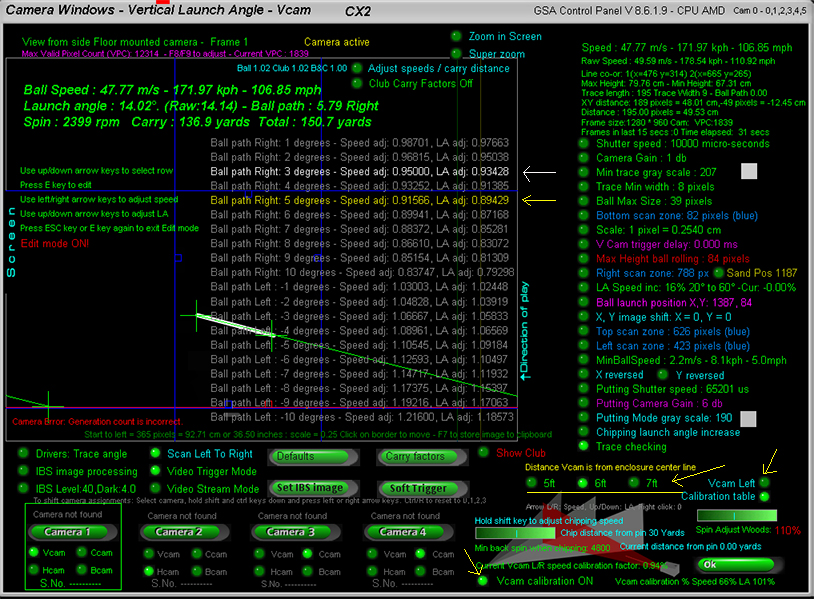Click the "Calibration table" option ON to view and edit the calibration tables

The yellow row shows the speed and LA adjustment made for the current shot

In the above example the ball speed was reduced to 91.566% of it's original speed and the LA reduced to 89.429% of it's original LA

The calibration is reducing (instead if increasing) both the speed and LA as the ball path is right - towards the camera - and thus the ball traces will appear larger.

The white row is the row selected by the edit cursor (Use up/down arrow keys to move).

Note that there are 3 sets of tables depending on the distance the Vcam is from the enclosure center line (i.e. 5 ,6 or 7ft)

Also note that the tables are reversed if the Vcam camera is mounted on the left side of the enclosure (Vcam left option ON)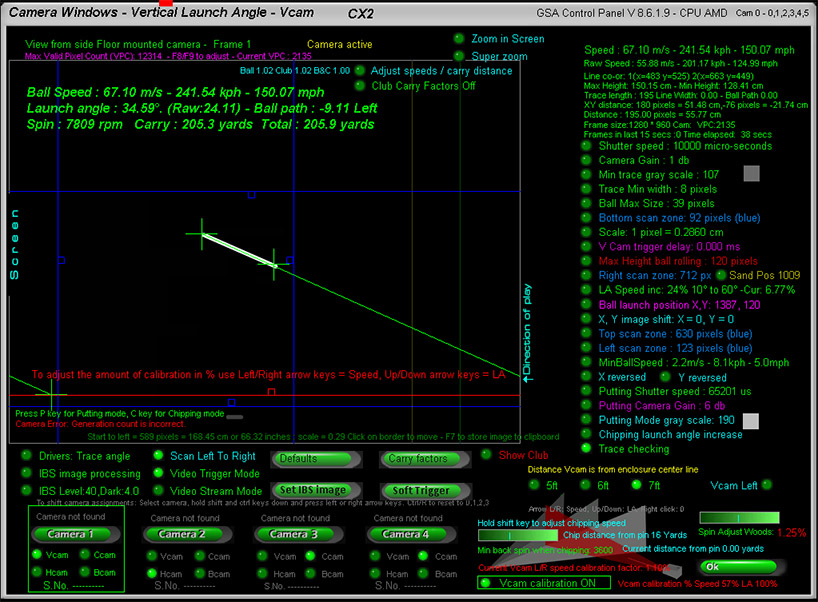To use this method of correction, switch the Vcam calibration ON in the Vcam window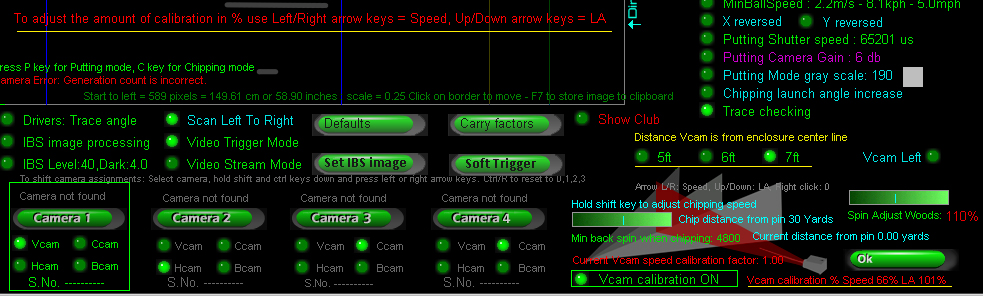Note that you can adjust the amount of calibration too

In the above example, speed calibration is reduced to 66% and LA calibration to 101%

Also note that the correct distance the Vcam is from the enclosure center line should be set

In the above example the distance is set to 7ft

In addition, ensure that if the Vcam camera is mounted on the left side of the enclosure, that the Vcam Left option is set to ON

Extended XY calibration

The new XY extended calibration expands this calibration to a 40 degree range (+/- 20 degrees)

for 6 launch vertical angle ranges of 10, 20, 30, 40, 50 and 60 degrees.

User defined percentage correction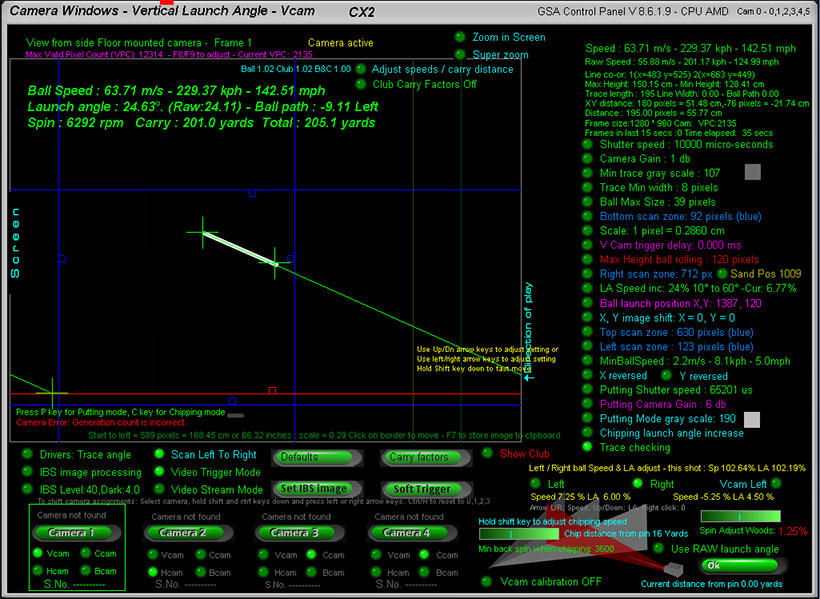• With the Vcam calibration switched OFF, a user defined percentage correction is used.
• In the above example, the camera is mounted on the right side of the enclosure ("Vcam left" option switched off)
• In this case the speed of the ball has to be decreased if the ball path is right (i.e. going towards the camera) and the speed increased if the ball is
• flying left (i.e. away from the camera).
• In the above example, the maximum speed increase for a ball flying left has been set to 7.25% and the vertical launch angle (LA) increase set to 6.00%
• For a ball flying right (i.e. towards the camera) the max speed decrease has been set to -5.25% and the LA increase set to 4.5%
• Note that these percentages are the maximum adjustment which is reached when the ball path is either 20 degrees right or 20 degrees left
• If the ball path is 10 degrees then half the adjustment is used and if just 5 degrees then only a 1/4 is used.

Hcam path correction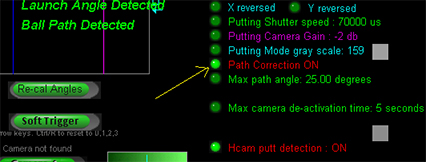To-date all calibration and linear adjustments where made for the Vcam camera which is responsible for ball speed and vertical launch angle calculations.

However, the Hcam ball path camera also requires either calibration or linear adjustment in order to calculate true ball path angles.

Calibration tests showed that the Hcam will only detect true ball path angles when the ball vertical launch angle is below 20 degrees.

As the vertical launch angle increases, the ball is getting nearer to the Hcam ceiling mounted camera and lens perspective distortions start to kick in.

Some examples are:

With a true ball path angle of 10 degrees left or right, the Hcam measured path without calibration was:

LA 20 degrees - path angle : 12 degrees

LA 30 degrees - path angle : 15 degrees

LA 40 degrees - path angle : 20 degrees

With a true ball path angle of 20 degrees left or right , the Hcam measured path without calibration was:

LA 20 degrees - path angle : 24 degrees

LA 30 degrees - path angle : 28 degrees

LA 40 degrees - path angle : 40 degrees

As can be seen, high lofted shots can result in ball paths being measured twice as much as they really are. i.e. a real path angle of 20 degrees, is measured as 40 degrees left or right without correction.

To correct this there's a new "Path correction" option in the Hcam window that linearly adjusts the path to it's correct value depending on the vertical launch of the ball.

An Hcam calibration table will be available shortly too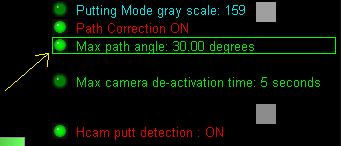BTW, if you have noticed that some high lofted shots weren't being detected, then it was probably due to the ball path being measured by the Hcam exceeded the "Max ball path" setting in the Hcam window.

Without path correction it would be very easy for the ball path to exceed the limit setting with high lofted shots and then the launch would be declared as invalid.

Chip speed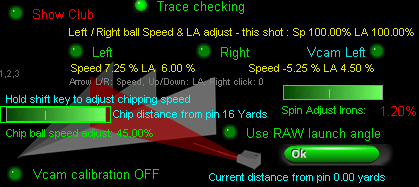• Even though the CP measures correct ball speed when chipping, these shots are often too long in the game software.
• i.e. the ball travels too far and skips off the green to the other side
• To correct this, you can set a ball speed adjustment percentage to reduce the speed of the ball.
• In the above example, the speed of the ball when chipping is reduced to 45.00% of the actual measured ball speed.
• This speed adjustment will only kick in when the system is in chipping mode and chipping mode will only kick in when
• the ball is not on the green
• but the distance the ball is to the pin is less than the user defined chip distance.

Chip distance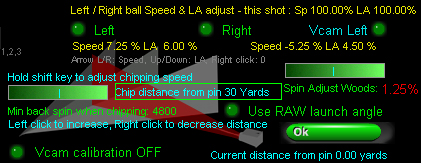To set the chip distance, hover the mouse cursor over the "Chip distance from pin" text and use left and right mouse clicks adjust the distance.

In the above example the distance to the pin where the chipping mode will be automatically activated is set to 30 yards.

"Min back spin when chipping"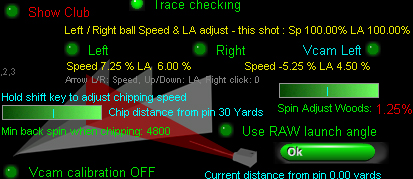Another major factor when chipping is the measured or calculated back spin

In order to prevent the ball from rolling straight off the green when chipping, the back spin should be quite high.

Use the slider bar to set the "Min back spin when chipping" to at least 4000 rpm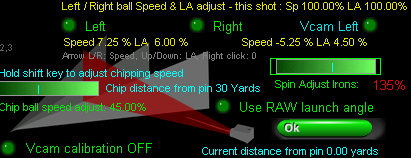When not using our ball spin cameras, spin rates are calculated from club and ball launch data

Use the spin adjust slider bar to increase or decrease the calculated spin rates.

In the above example, calculated spin rate for all irons has been increased by 135%

Note that spin rate adjustment settings are separate for irons and woods. Hold the shift key down to select irons, otherwise woods are selected.

Hcam path correction

Some small tweeks were made to the Hcam path correction today and the new 7ft calibration table was made with Hcam correction ON.

5ft and 6ft tables didn't use the Hcam correction so will be redone mext week. In the meantime use the 7ft table even if your Vcam is closer or further away.

Chipping mode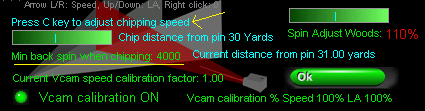When in Chipping Mode, the "min backspin when chipping" slider bar turns into .... "chip ball speed adjust"and "Launch Angle inc" turns into "Chipping launch angle inc"

Note "Launch Angle inc" is not required when using "Extended Calibration".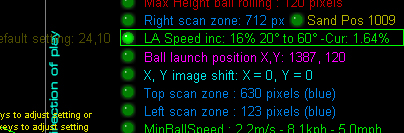• In addition to ball speed adjustments for balls flying left or right, there's also ball speed adjustments related to the launch angle of the ball.
• The reason for this is because as the ball flies higher with higher launch angles,
• the ball is getting further away from the floor mounted camera and thus the trace length appears smaller
• which in turn results in lower speed measurements.
• To correct this an "LA Speed" adjustment based on the launch angle of the ball can be set.
• In the above example, a max ball speed increase of 16% is set over an LA range of 20 to 60 degrees.
• This means that if the ball LA is below 20 degrees then no adjustment will be made but if higher then a percentage of the 16% will be applied to the ball speed.
• e.g. If the LA is 60 degrees then the full 16% will applied, if 40 degrees (i.e. halfway between 20 and 60 degrees) then there will only be an 8% (half of 16%) increase.
• (BTW the "Cur:" means Current percentage increment used based on the current launch angle of a shot)
• Note that if not using this adjustment, high lofted shots may carry too short while woods and long iron carry distances are OK
• Also note that this adjustment can be reversed. i.e. the percentage adjustment can be minus and thus the ball speed will be decremented instead of
• increased with increasing launch angles
• Launch angle adjustments (only used when calibration is OFF)
• All the calibration work done recently has shown that un-calibrated measured launch angles are far lower than what they really are as viewed by the cameras. Usually around 25 to 30% less
• For those that prefer linear adjustments over calibration we've added a linear launch angle adjustment in the Vcam window in this version of the CP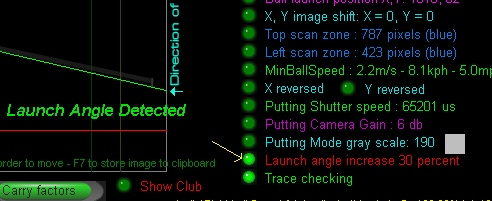Just click multiple times on the new "Launch angle increase" option to set the LA increment to be 0, 5, 10, 15, 20,25, 30 or 40%

Scaling factor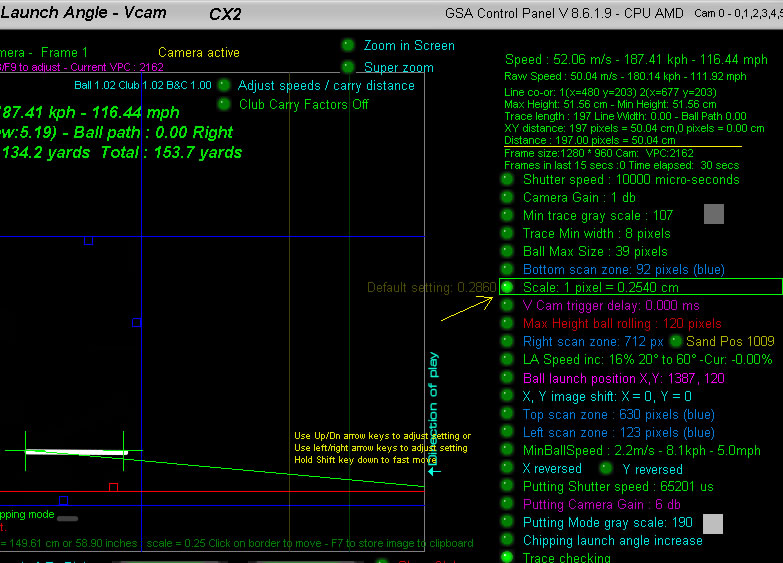• The CP calculates ball speed by the length of the ball trace of the shot.
• A ball trace of a fast moving ball is achieved by using relatively long camera exposure times
• In the above example the exposure time (or shutter speed) is set to 10000 micro seconds = 0.01 seconds
• The length of the trace in the camera image is in pixels so this length (197 px in the above example) has to be converted to
• real world length units before it can be used in the speed formula v = d/t
• This conversion is done by the user defined "Scaling factor"
• In the above example the "Scale" is set to 0.2450 which means each pixel in the image is equivalent to 0.2450 cm
• In the above the trace length in pixels is 197 which after conversion is 50.04 cm (i.e. 197 x 0.2450 = 50.04)
• After this conversion we can use v = d/t = 0.5/0.01 = 50 meters per second
• Setting the base scaling factor
• Most just use the default setting of 0.2860 but if you would like to be more exact then the following method should be used.
• 1. Place a length of white tubing or white pole of a known length in the field of view of the Vcam camera
• 2. Click the Soft Trigger button to grab a new image in the Vcam window
• 3. Adjust the scale factor so that the "Distance" is the same as the known length of the pole.
• In the above example the tube length is 50 cm and the "Scale" is set to 0.2450 so that the "Distance" is also 50 cm

Individual club carry factorsYou can also add speed / carry factors for each club separately in this window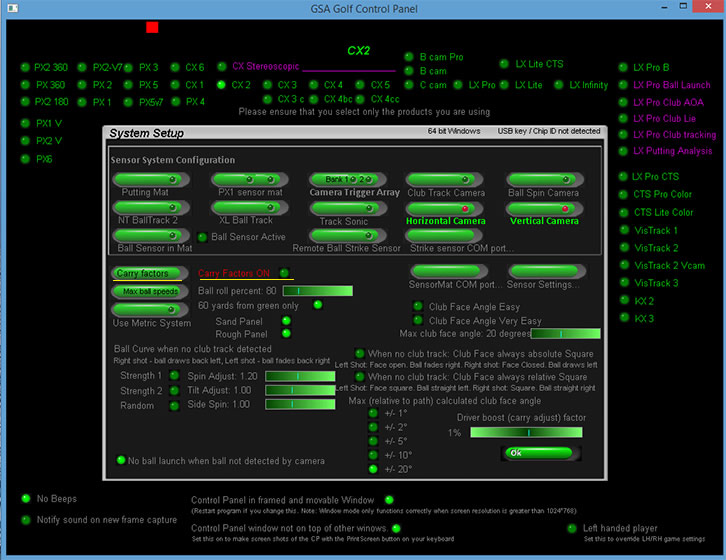Click the "Carry Factors" button in the CP's Setup window to access this carry factors window.

Note that the "Carry Factors" option has to be set ON in order for them to take effect

There's also a "Driver boost" factor in the Setup window that just adjusts ball speeds for Drivers

Adjust carry and spin directly in the Vcam window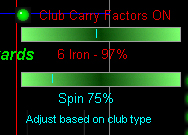There's a new slider bar in the Vcam window that allows users to adjust the LA based carry and spin factors directly in the Vcam window and thus see the results of carry adjustment immediately.

New speed & spin adjustments based on launch angles as well as club typesIn addition to the carry & spin factors based on the club being used, there is now a carry (ball speed) factor and spin rate factor based on ball launch angles.

This should be more accurate than the club based method as it cannot be what the launch angle is based on the club selection.

Note that these carry and spin factors are either club or LA based and not both together. If "Carry factors" are switched OFF then neither will be used.

The third column in the window is used to set spin rate factors for specific clubs or launch angles. Again, if "Carry factors" are switched OFF then these spin factors will not be used.Also note that the standard LA speed increase or decrease is still active in the Vcam window when selecting carry factors ON.

Click the above image and scroll down to Q 16 to read more about standard LA speed adjustments.

V and H cam

126 step extended XY Calibration

for V and H cam camera setups

The calibration method here is to use a white tube of a known length on a pole that represents a ball trace at a constant speed which is then placed at various vertical and horizontal angles.

The object of the calibration is to ensure that the length of the trace (i.e. white tube) remains constant at all angles and the launch angle remains constant for the particular tilt of the pole as measured by an inclination meter

Note that there is a standard factory set extended calibration for XY camera setups but if you'd like calibrate your system yourself then this is how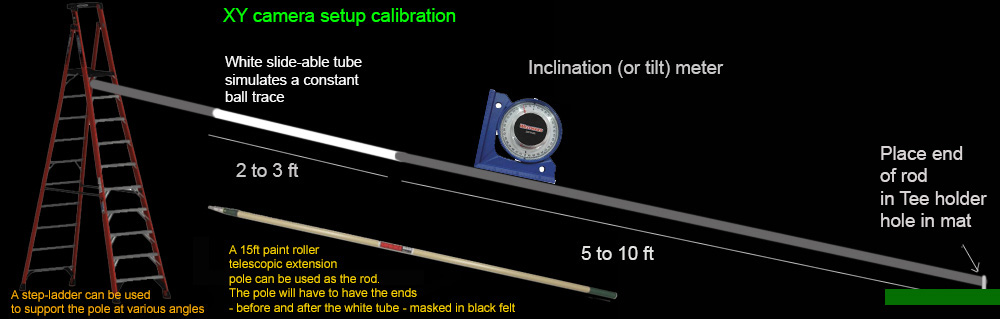The V and Hcam calibration method involves using a black or dark long rod with a movable white plastic tube or paper roll attached to one end

and pinned down at the other end with a swivel mounted on a base plate at the usual ball hitting position on the hitting mat.Place the end of the rod in the hitting mat's tee holder hole.

This represents the ball's initial position on the ground.

The rod is tilted upwards in 10 degree steps from 10 degrees to 60 degrees to simulate various launch angles of the ball

and moved both left and right in 2 degree steps to simulate ball path from 20 degrees left to 20 degrees right.

The total number of entries is then 21 x 6 = 126

A tilt meter is used to measure the real angle of the rod and the Hcam is used to measure the ball path.

Slide the white tube up or down as necessary to keep it in the same FOV of the camera with every step. This simulates the same trigger point.

With each step, capture new V and H cam images of the rod by pressing the Enter key.

Pressing the Enter key on your PC's keyboard sends "Soft trigger" instructions to both the V and H cams.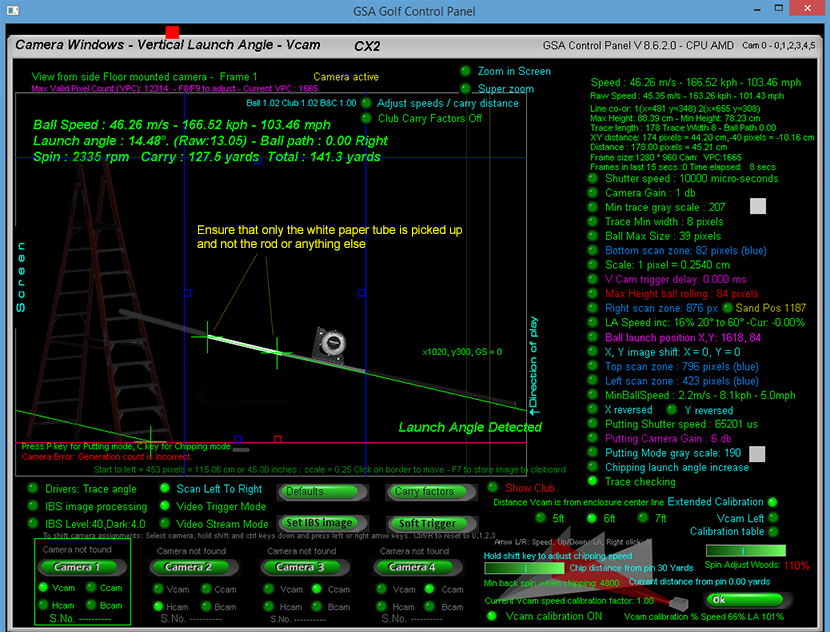Use blocks of some kind (e.g. step ladder, stools or chairs etc) to support the end of the rod to get the desired vertical angles.Use the Hcam to check the desired ball path

Ensure that the "Ball Launch Position" has been set correctly in the Hcam window.

Start at Vertical angle of 0 degrees and zero degree path and set the scaling factor so that the distance measured in the Vcam is the same as the actual length of the white part of the rodIn the above case, the white tube is 50 cm long and the length measured by the camera in pixels is 197 px

The scaling factor is then set to a value that converts the length measured in pixels to 50cm. In this case: 0.254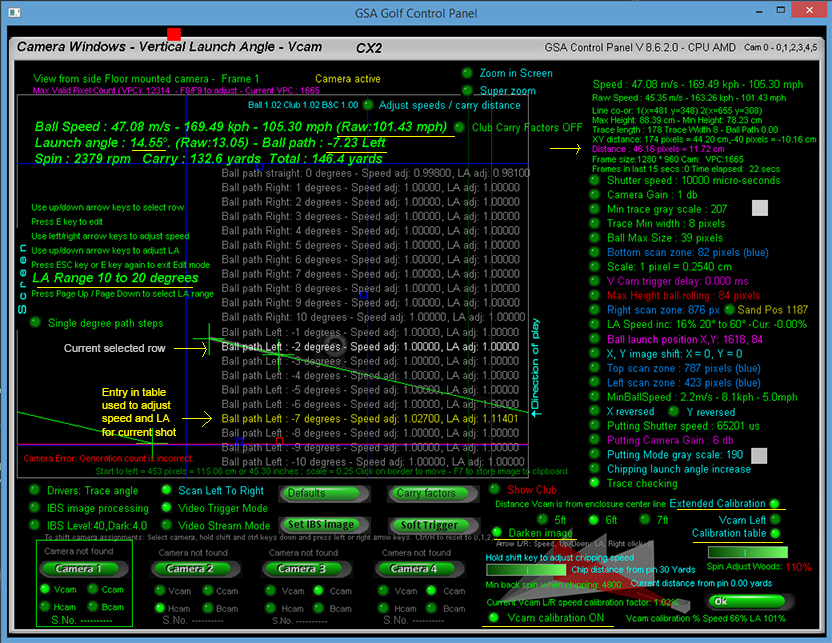• The above image shows the new extended calibration table.
• There are 6 pages for launch angles (LA) from 10 to 60 degrees in 10 degree steps
• Each page consists of 21 LA and Speed adjustment rows for ball paths in the range of -10 to + 10 degrees in single degree steps
• (You can also set the path range to -20 to +20 degrees by switching the path steps to 2 degrees)
• During the calibration process a ball launch angle, ball speed and ball path will be calculated from the pole
• Use the page up and page down keys to select the LA range of the shot
• In the above example LA was 14.55 degrees so the range 10 to 20 is selected
• The RAW ball path measured by the Hcam in this example is -7.23 degrees left.
• The yellow text cursor will then select the nearest entry in the table to -7 degrees.
• You should then see the entry in the table that is used to adjust the LA and Speed for this particular shot (or pole placement)
• Move the current row cursor (shown in white text) to the active entry (shown in yellow text) and press the "E" key to edit
• Adjust the speed (with the left and right arrow keys on your keyboard)
• and LA correction factors (up and down arrow keys)
• until the length of the white rod length measured in the Vcam window is the same as
• the real length (shown as Distance or Trace Length ) in this color at the top right corner of the screen
• and the measured Launch angle (shown in large green text at the top of the screen) is the same as the real launch angle as read from the tilt meter.
• Press the ESC key to exit Edit Mode
• Continue the process until all 120 LA and Path position entries have been made.
• Note that when calibrating, ensure that all other speed and LA adjustments are off.
• i.e. Carry factors are set to OFF.and LA speed inc / dec is set to zero percent

as these adjustments will no longer be required after calibration

Note that there are a number of new features in the Vcam window.

1. Raw speed is now shown. Raw speed is the speed calculated from the camera without any adjustments or calibration

2. A Darken image option is now available that darkens the Vcam image so thet the calibration table is more readable.

3. Calculated trace length distance is now shown in a pink shade so that it stands out more

Auto calibration• With the auto calibration mode ON, the calibration process will automatically set the trace distance and LA corrections for you.
• All you need to do is place the pole at the -20 to +20 degree path angles in the Hcam window - which will automatically
• be set in video stream mode - then switch to the Vcam.
• After switching to the Vcam window, the adjustments will be automatically calculated and and you're ready to go back to the
• Hcam window again for the next path angle and/ or launch angle.
• Total calibration time in this mode should be less than 30 minutes for all 126 launch angle and path calibrations
• Ensure the following when using Auto Calibration
• 1. Vcam calibration option is ON
• 2. Extended calibration option is ON
• 3. The base trace length is set to the real length of the white tube
• 4. Auto Calibration adjust option is ON
• 5. The LA range is set to the vertical angle of the white tube
• 6. The Vcam calibration percent is set to 100 pecent for both speed and LA
• Calibration procedure
• 1.Start with LA range 0 to 10 degrees and switch to the Hcam window
• You will see that the Hcam is already in video stream mode.
• 2. Move the pole to zero degrees path. The path angle of the pole will automatically be shown in degrees as you move the pole left and right.
• And the vertical angle to 10 degrees or a little less by placing a support under the pole
• 3. Switch to the Vcam. You should now see that the LA is automatically set to 10 degrees and trace length to your base trace length.
• 4. Switch back to the Hcam and move the pole right until the Hcam shows 2 degrees right
• 5. Switch back to the Vam
• Repeat this process for all degrees right and left i.e. moving in 2 degree steps from 0 degrees path to +20 degrees right
• and from zero to -20 degree left
• Once complete, adjust the pole to 20 degrees and use the page up/down keys to go to the 10 to 20 degree range.
• Start the left right pole path procedure again.
• Repeat for vertical pole angles of 10, 20, 30 ,40 and 60 degrees
• The end result of this calibration should be absolute consistency across the entire vertical and horizontal range
• from 0 to 20 degree ball paths left and right and launch angles from 0 to 60 degrees
• Auto calibration mode - now even faster
• To speed up calibration even further, you can switch between the Vcam and Hcam by simply pressing the "Space" bar on the keyboard.
• This saves you the time it would normally take to switch cameras with the mouse.
• And instead of having to look at the screen to see if the auto calibration worked for each angle step every-time, there's now an audible beep that tells you all is OK.

Calibration Example• The above shows a typical calibration table
• In this example, the pole was angled at 30 degrees vertically and 20 degrees left.
• The white tube on the pole - i.e. the part that represents the ball trace - was 56cm in length
• Without calibration the launch angled measured by the camera was only 23.41 degrees. The correction value was automatically
• set to 1.28 in order to measure the true launch angle of 30 degrees
• The trace length measured by the camera was smaller than the 56 cm so the correction value was automatically set to 1.032.
• The trace length dictates the speed of the ball and before calibration the measured speed (Raw speed) was only 121.45 mph.
• After calibration. speed was up to 133.4 mph.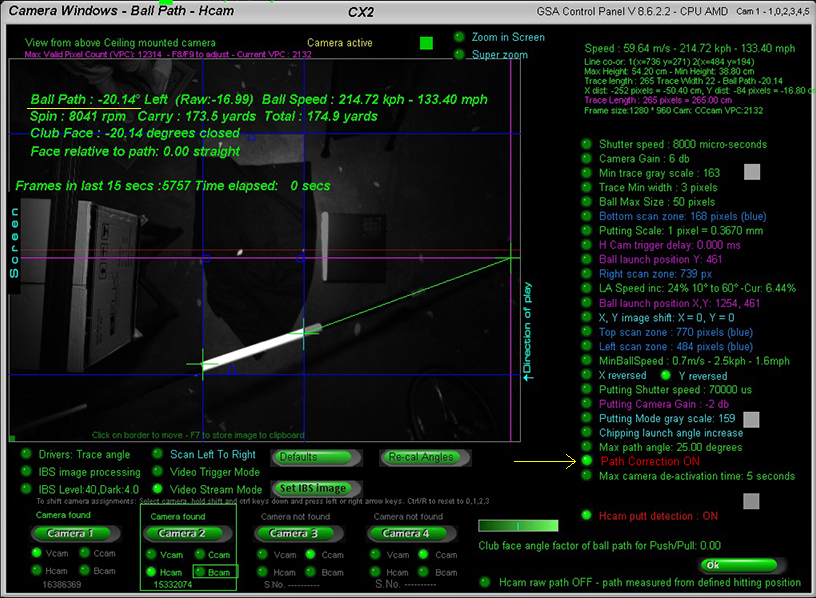Here the Hcam is being used to measure the ball path angle during the calibration process

Note that Hcam "ball path correction" has to be switched ON in order to use the Hcam to measure ball paths accurately

If not using the Hcam to measure the path of the pole then a plum line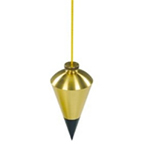suspended from the pole beginning over a large protractor will have to be used to measurre exact pole paths

Calibration process tips

• 1. When setting the pole vertical angle, ensure that the angled measured with the tilt meter is no greater than the selected vertical range.
• e.g. when selecting a launch angle range of 10 to 20 degrees, set the pole to 20 degrees and no more
• when selecting a launch angle range of 20 to30 degrees, set the pole to 30 degrees and no more and so on
• 2. When moving the pole left and right in the 2 degree steps to simulate the ball path, ensure that the complete white part of the pole is detected in the Vcam
• and that the measured raw vertical launch angle stays below the LA range max.
• e.g. if the range is 20 to 30 degrees then the raw angle (i.e. the angle without calibration ) is no greater than 30 degrees
• 3. With every step, ensure you hear the audible beep when pressing the space bar to get back to the Vcam from the Hcam
• Current CP calibration updates include:
• 1. Pressing the Z key resets the calibration on the active row to zero calibration (i.e. 1.0 correction factor)
• 2. Pressing the ESC key closes the calibration table
• 3. An index check is made with each auto calibration step to ensure that the correct table is being accessed
• No beep is played if index is not in the correct range or when any other error occurs during the calibration process

Factory calibration table set

All current GSA Golf Control Panel versions and updates now include a factory set camera calibration table

that negates any user requirement to calibrate the cameras themselves.

The table consists of 3 calibration tables each consisting of 126 entries

for various distances the Vcam is from the center line

Vcam distances from the center line are 5ft, 6ft and 7ft.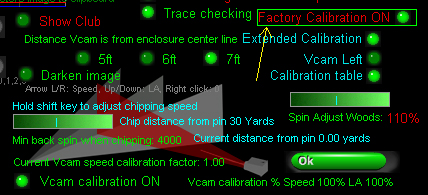Note that the calibration table "calibLRex.csv" is placed in the "C:\Program Files (x86)\GSAControlPanel\data" folder when you download the latest CP update.

In order to use it, the new "Factory Calibration" option must be on.

Any user calibration tables won't be overwritten when this option is on but if you would like to edit the factory tables then switch the "Factory Calibration" option OFF again before editing.

The table will then be automatically saved to the "C:Users\Public" folder.

Calibration factors used now displayed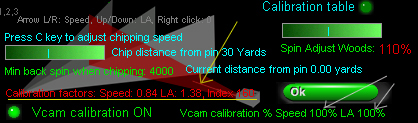The calibration factors used for the current shot are now displayed in the Vcam window.

These are Speed adjustment, Launch Angle (LA) adjustment and the index number into the calibration table.

In the above example, the raw speed of the ball was reduced to 84% and the raw LA increased by 38%

Extended calibration "Scale" factor = 0.2780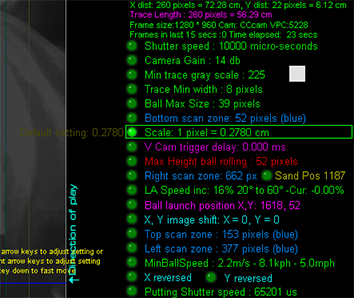Note that the Scale factor for the calibration tables was set to 1 pixel = 0.2780.

This scale factor used with the calibration tables should result in exact ball speed measurements across the entire 0 - 60 degree launch angle and +/- 20 degree path range.

Increasing or decreasing this scale will result in decreased or increased measured ball speed across the entire range should you care to change it.

Don't forget !

If your Vcam is mounted on the left side of the enclosure, ensure that the "Vcam Left" option is ON

otherwise your calibration will be reversed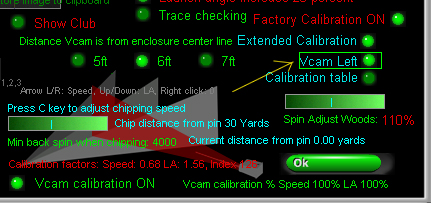Also don't forget to set the distance your Vcam is from the enclosure center line

Currently distances are 5ft, 6ft and 7 ft.

If your Vcam is over 7 ft away from the center line then just use 7ft setting for now.

Other distances will be available later this yearAlso don't forget to set the amount of calibration to 100% for both speed and LA to start with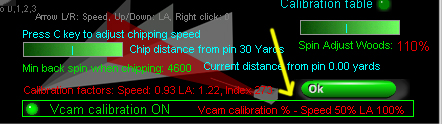Later you can reduce or increase the amount of clibration for both Speed and LA to suit

In the above example the speed calibration was reduced to 50% so that any ball speed adjustments for ball hit left or right will be 50% less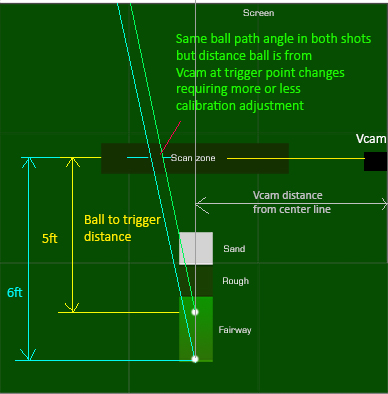Note that the calibration tables where made with a ball to trigger distance of 5ft.

Later next month we'll have exact speed calibration percentages for various other ball to trigger distancesAlso don't forget to set "Extended Calibration" ON and "Factory calibration" ON

Note that these are default ON with the new CP releases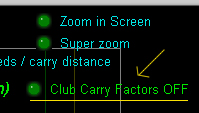And finally, don't forget to switch "Carry factors" OFF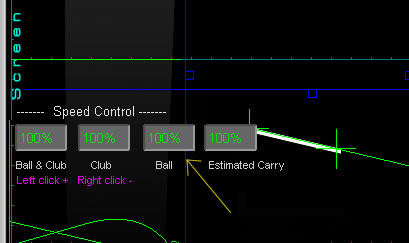and "Ball speed controls" to their default of 100%